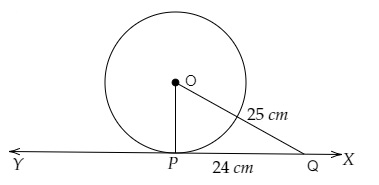# If the tangent at a point P to a circle with centre O cuts a line through O at Q such that $PQ = 24\ cm$ and $OQ = 25\ cm$. Find the radius of the circle.

Given:

The tangent at a point P to a circle with centre O cuts a line through O at Q such that $PQ = 24\ cm$ and $OQ = 25\ cm$.

To do:

We have to find the radius of the circle.
Solution:$PQ = 24\ cm$ and $OQ = 25\ cm$

Let $XY$ be the tangent to the circle at $P$.

$OP\ perp\ XY$

In right angled triangle $OPQ$,

By Pythagoras theorem,

$OQ^2= OP^2+ PQ^2$

$(25)^2 = OP^2 + (24)^2$

$OP^2= 625 - 576$

$= 49$

$= 7^2$

Therefore,

$OP = 7\ cm$

The radius of the circle is 7 cm.

Updated on: 10-Oct-2022

29 Views